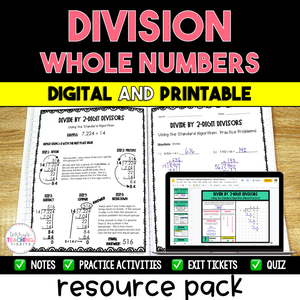# Division of Whole Numbers Resource Bundle - Digital & Printable

• \$25.00
Unit price per
• Save \$3
Shipping calculated at checkout.

This digital and printable resource pack contains everything you need to teach Division of Whole Numbers: detailed notes, student practice pages/activities, exit tickets, and a quiz.

You will successfully help your students learn how to use mental math, the standard algorithm, and partial quotients to divide by 1-digit and 2-digit divisors.

9 Mini-Lessons included.

Standards:  5.NBT.6

This resource is also part of my 5th Grade Math Year-Long Curriculum Bundle.

Teaching Suggestions:

1. Print the notes page and practice problems page on half sheets of paper for students to tape into composition books. This makes a great reference tool. Introduce the skill by going over the example problem and then guide the students through the sample problems, allowing students to demonstrate the problem on an interactive whiteboard.

(You can pull the PDF up on an interactive screen and annotate over it with programs such as Promethean’s ActivInspire. You can also use a document camera to project a student’s notebook)

2. Allow students to work independently on one of the practice pages and check on their progress.

3. Check for understanding/mastery either at the beginning or end of class with an exit ticket.

4. If time allows, use a second day to reinforce the skill with the second practice page and exit ticket before moving on to the next skill.

5. When all of the mini-lessons have been taught, assess for mastery using the quiz.

## What You Get

9 Mini-Lessons

Digital Version:

• Notes and Practice in Google Slides
• Tutorial Videos included in each mini-lesson
• Exit Tickets and Quiz in Google Forms

Division with Mental Math

• detailed notes with sample problems
• 3 practice activities
• 3 exit tickets

Division: 1-Digit Divisors {Standard Algorithm}

• 3 mini-lessons
• standard algorithm
• notes with sample problems
• practice activity
• zeros in the quotient
• notes with sample problems
• practice activity
• remainders
• notes with sample problems
• practice activity
• 2 mixed reviews of the 3 types of problems above
• 6 exit tickets

Division: 1-Digit Divisors {Partial Quotients}

• detailed notes with sample problems
• 2 practice pages
• 4 exit tickets

Division: 2-Digit Divisors {Standard Algorithm}

• 3 mini-lessons
• standard algorithm
• notes with sample problems
• practice activity
• zeros in the quotient
• notes with sample problems
• practice activity
• remainders
• notes with sample problems
• practice activity
• 2 mixed reviews of the 3 types of problems above
• 6 exit tickets

Division: 2-Digit Divisors {Partial Quotients}

• detailed notes with sample problems
• 2 practice pages
• 4 exit tickets

Quiz